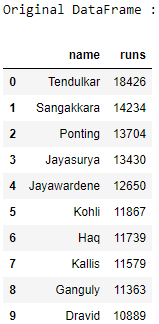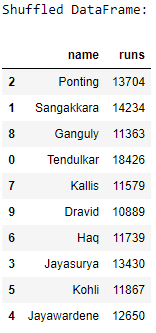# Pandas – How to shuffle a DataFrame rows

Let us see how to shuffle the rows of a DataFrame. We will be using the `sample()` method of the pandas module to to randomly shuffle DataFrame rows in Pandas.

Algorithm :

1. Import the `pandas `and `numpy `modules.
2. Create a DataFrame.
3. Shuffle the rows of the DataFrame using the `sample()` method with the parameter `frac` as 1, it determines what fraction of total instances need to be returned.
4. Print the original and the shuffled DataFrames.

 `# import the modules ` `import` `pandas as pd ` `import` `numpy as np ` ` `  `# create a DataFrame ` `ODI_runs ``=` `{``'name'``: [``'Tendulkar'``, ``'Sangakkara'``, ``'Ponting'``, ` `                      ``'Jayasurya'``, ``'Jayawardene'``, ``'Kohli'``, ` `                      ``'Haq'``, ``'Kallis'``, ``'Ganguly'``, ``'Dravid'``], ` `            ``'runs'``: [``18426``, ``14234``, ``13704``, ``13430``, ``12650``, ` `                     ``11867``, ``11739``, ``11579``, ``11363``, ``10889``]} ` `df ``=` `pd.DataFrame(ODI_runs) ` ` `  `# print the original DataFrame ` `print``(``"Original DataFrame :"``) ` `print``(df) ` ` `  `# shuffle the DataFrame rows ` `df ``=` `df.sample(frac ``=` `1``) ` ` `  `# print the shuffled DataFrame ` `print``(``"\nShuffled DataFrame:"``) ` `print``(df) `

Output :My Personal Notes arrow_drop_upCheck out this Author's contributed articles.

If you like GeeksforGeeks and would like to contribute, you can also write an article using contribute.geeksforgeeks.org or mail your article to contribute@geeksforgeeks.org. See your article appearing on the GeeksforGeeks main page and help other Geeks.

Please Improve this article if you find anything incorrect by clicking on the "Improve Article" button below.

Article Tags :

Be the First to upvote.

Please write to us at contribute@geeksforgeeks.org to report any issue with the above content.